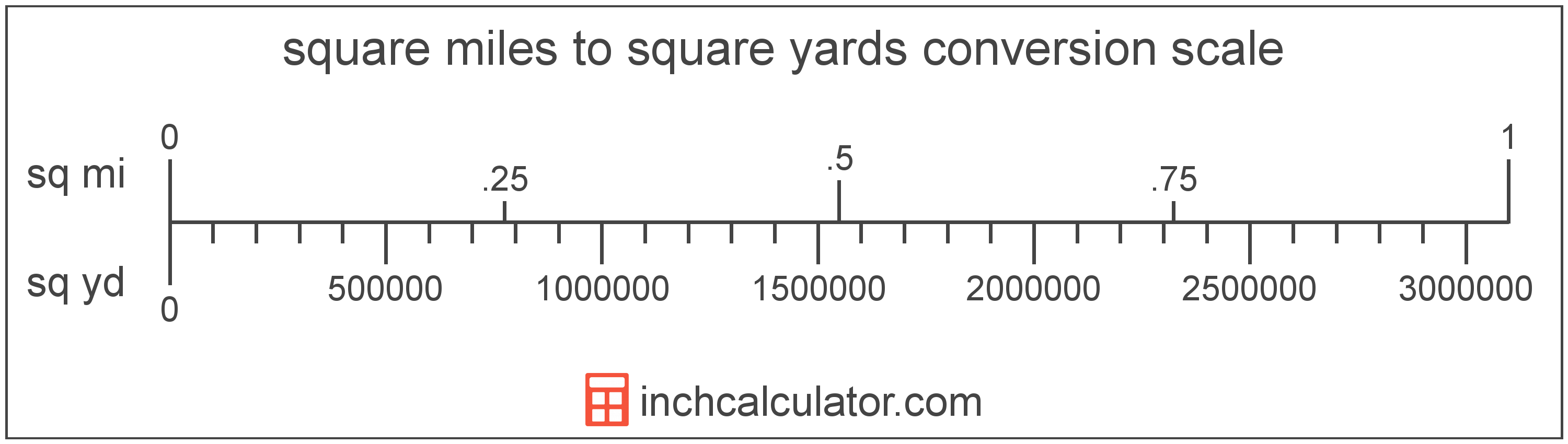# Square Miles to Square Yards Converter

Enter the area in square miles below to get the value converted to square yards.

Results in Square Yards:1 sq mi = 3,097,599.999598 sq yd

Do you want to convert square yards to square miles?

## How to Convert Square Miles to Square Yards

To convert a measurement in square miles to a measurement in square yards, multiply the area by the following conversion ratio: 3,097,599.999598 square yards/square mile.

Since one square mile is equal to 3,097,599.999598 square yards, you can use this simple formula to convert:

square yards = square miles × 3,097,599.999598

The area in square yards is equal to the area in square miles multiplied by 3,097,599.999598.

For example, here's how to convert 5 square miles to square yards using the formula above.
square yards = (5 sq mi × 3,097,599.999598) = 15,487,999.997991 sq yd### How Many Square Yards Are in a Square Mile?

There are 3,097,599.999598 square yards in a square mile, which is why we use this value in the formula above.

1 sq mi = 3,097,599.999598 sq yd

## What is a Square Mile?

One square mile is equal to the area of a square with sides that are each 1 mile long. One square mile is roughly equal to 2.59 square kilometers or 640 acres.

The square mile is a US customary and imperial unit of area. Square miles can be abbreviated as sq mi, and are also sometimes abbreviated as mi². For example, 1 square mile can be written as 1 sq mi or 1 mi².

## What is a Square Yard?

One square yard is equivalent to the area of a square with sides that are each 1 yard in length. One square yard is roughly equal to 9 square feet or 0.836127 square meters.

The square yard is a US customary and imperial unit of area. A square yard is sometimes also referred to as a square yd. Square yards can be abbreviated as sq yd, and are also sometimes abbreviated as yd². For example, 1 square yard can be written as 1 sq yd or 1 yd².

You can use a square yards calculator to calculate the area of a space if you know its dimensions.

## Square Mile to Square Yard Conversion Table

Table showing various square mile measurements converted to square yards.
Square Miles Square Yards
0.000001 sq mi 3.0976 sq yd
0.000002 sq mi 6.1952 sq yd
0.000003 sq mi 9.2928 sq yd
0.000004 sq mi 12.39 sq yd
0.000005 sq mi 15.49 sq yd
0.000006 sq mi 18.59 sq yd
0.000007 sq mi 21.68 sq yd
0.000008 sq mi 24.78 sq yd
0.000009 sq mi 27.88 sq yd
0.0000001 sq mi 0.30976 sq yd
0.000001 sq mi 3.0976 sq yd
0.00001 sq mi 30.98 sq yd
0.0001 sq mi 309.76 sq yd
0.001 sq mi 3,098 sq yd
0.01 sq mi 30,976 sq yd
0.1 sq mi 309,760 sq yd
1 sq mi 3,097,600 sq yd